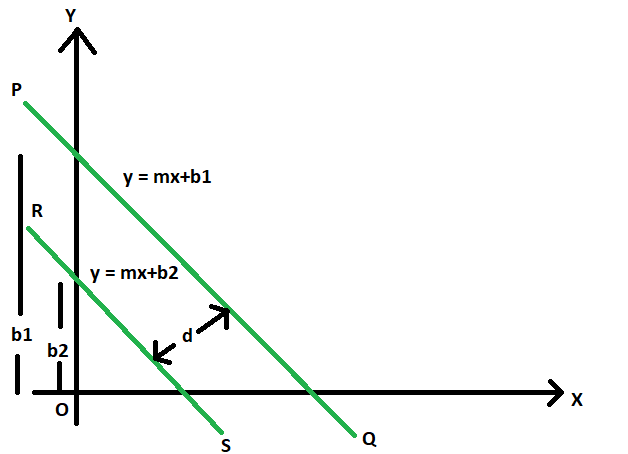Related Articles

# Distance between two parallel lines

• Last Updated : 18 Mar, 2021

Given are two parallel straight lines with slope m, and different y-intercepts b1 & b2.The task is to find the distance between these two parallel lines.
Examples:

Input: m = 2, b1 = 4, b2 = 3
Output: 0.333333

Input: m = -4, b1 = 11, b2 = 23
Output: 0.8Approach:

1. Let PQ and RS be the parallel lines, with equations
y = mx + b1
y = mx + b2

2. The distance between these two lines is the distance between the two intersection points of these lines with the perpendicular line.Let that distance be d

3. So, equation of the line perpendicular to PQ and RS can be
y = -x/m

4. Now, solving the perpendicular line with PQ and RS separately to get the intersecting points (x1, y1) & (x2, y2), we get,

5. From PQ
y = mx + b1
y = -x/m
(x1, y1) = ( -b1*m/(m^2 + 1), b1/(m^2 + 1))

6. From RS
y = mx + b2
y = -x/m
(x2, y2) = ( -b2*m/(m^2 + 1), b2/(m^2 + 1))

7. So, d = distance between (x1, y1) and (x2, y2)Below is the implementation of the above approach

## C++

 // C++ program find the distance// between two parallel lines #include using namespace std; // Function to find the distance// between parallel linesdouble dist(double m, double b1, double b2){    double d = fabs(b2 - b1) / ((m * m) - 1);    return d;} // Driver Codeint main(){    double m = 2, b1 = 4, b2 = 3;    cout << dist(m, b1, b2);    return 0;}

## Java

 // Java program find the distance// between two parallel linesclass GFG{     // Function to find the distance// between parallel linesstatic double dist(double m,                double b1, double b2){    double d = Math.abs(b2 - b1) /                    ((m * m) - 1);    return d;} // Driver Codepublic static void main(String[] args){    double m = 2, b1 = 4, b2 = 3;     System.out.println(dist(m, b1, b2));}} // This code is contributed by Code_Mech.

## Python3

 # Python3 program find the distance# between two parallel lines # Function to find the distance# between parallel linesdef dist(m, b1, b2):    d = abs(b2 - b1) / ((m * m) - 1);    return d; # Driver Codedef main():    m, b1, b2 =2,4, 3;    print(dist(m, b1, b2));if __name__ == '__main__':    main() # This code contributed by PrinciRaj1992

## C#

 // C# program find the distance// between two parallel linesusing System; class GFG{     // Function to find the distance// between parallel linesstatic double dist(double m,                   double b1, double b2){    double d = Math.Abs(b2 - b1) /                      ((m * m) - 1);    return d;} // Driver Codepublic static void Main(){    double m = 2, b1 = 4, b2 = 3;    Console.Write(dist(m, b1, b2));}} // This code is contributed by Akanksha Rai

## PHP

 

## Javascript

 
Output:
0.333333

Attention reader! All those who say programming isn’t for kids, just haven’t met the right mentors yet. Join the  Demo Class for First Step to Coding Coursespecifically designed for students of class 8 to 12.

The students will get to learn more about the world of programming in these free classes which will definitely help them in making a wise career choice in the future.

My Personal Notes arrow_drop_up